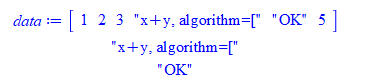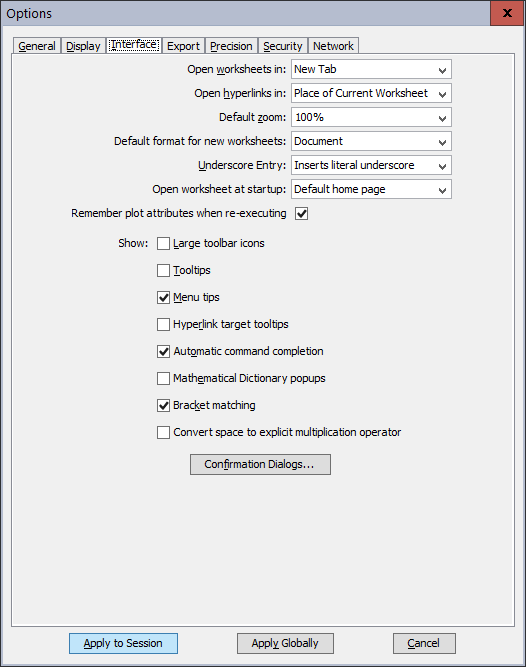## i'm receiving this error "Error, invalid input: d...## How to import CSV (comma delimited) text file with...

I am exploring the idea of porting some of my functions to Maple from Mathematica. But I just found a big problem in importing comma delimited data that contains a string within a string. This is not a problem being imported in Mathematica, but I need help to do same thing in Maple.

I tried ImportMatrix (as suggested in https://www.mapleprimes.com/questions/221509-How-To-Read-Lines-From-File-With-Quoted-Strings and also tried Import then convert and in both cases Maple does not read the full string in the field. I will show simple example

This file, called data.txt

`1,2,3,"x+y, algorithm=["123"]","OK",5`

It has 6 fields (since comma seperated.

But when importing it, Maple reads the 4th field above as "x+y, algorithm=[" and drops the rest 123"]"

Here is the code

```#currentdir("C:\\bug"); #change as needed
data:=ImportMatrix("data.txt", source=csv);
print(data(4));
print(data(5));
```

This is the outputI also tried

```data := Import("data.txt",format="CSV");
data := convert(data,Matrix):
print(data(4));
print(data(5));
```

Same output as above.

Compare the output in Mathematica. Using the same file as input. It automatically escapes the embedded strings and reads the full field OK

```SetDirectory[NotebookDirectory[]];
data = First@Import["data.txt", "Table", "FieldSeparators" -> {","}, "Numeric" -> True]

{1, 2, 3, "x+y, algorithm=[\"123\"]", "OK", 5}

data[]

"x+y, algorithm=[\"123\"]"

```

What would be the right way in Maple to import such files without losing part of the field?

The file has mixed strings and non strings, just like this example shows, and each file fields are seperated by comma, but I need to be able to read fields that can contain embedded strings in them automatically.

I am using Maple 2020. I think I might have to forget about import and try to do it using lower level code using scanf, or may be there is better method using Filetools package? but thought to check first if I am overlooking some option.

## very annoying problem in user interface. Losses br...

I depend alot on bracket matching to help me during writing code.

Maple suddenly losses bracket matching at some lines. I think it related to using strings with "\\" in them.

I make sure I have bracket matching onI use worksheet mode (corrected), Maple 2020 under windows 10.

I made small video. Notice how the brackets do not match on the second line there.Do you see how the brackets do not match on that second line there? This movie below shows what happens when I remove "\\string" from the line. Now bracket match starts to work again.This is a serious problem for me. Any suggestions what to try to fix it and what can cause it?. Once bracket matching is lost, it is lost all the way to the rest of the code below the location where it started to lose first time.

```restart;
if length(data_last(k,8))>300 then
fprintf(file_id,"%s\n","\\footnotesize");
fi;
```

I think I have to find different editor to use and write the code to .mpl file since I have lots of such code and I need to have bracket matching working.

## Is it possible to calculate the Riemann Tensor for...

I am interested in trying to find the Kretschmann Scalar of certain metric, where the line element in given bellow as well as conditions on the indices.I am wondering if there is any possible way to compute the Riemann tensor components just using indices with the physics package or the tensor package. I have used them both before but for metrics which are explicitly defined.

Thank you.

## Physics Commutator does not sum over repeated indi...

Hello,

 >(1)
 >I have the following issue.

The way it is set at the moment, works kinda in that the final result is 0, which I tried to check.

However, the expression L2L2X is not fully Simplified, since the term

-(4*X[a])*X[b]^2*P[a]*P[k] + (4*X[b]^2)*X[f]*P[f]*P[k]

is actually zero. I suppose that he doesn't manage because of the different indices "a" and "f" here. How can this be fixed?

When I comment out "Define" and use "Coordinates(X,P)" instead, it doesn't work. Seems to give the wrong result (Difference is not zero).

## How to stop Maple from adding new variables to dif...

Hey,

I'm trying to differentiate a function but whenever I do so, it adds new variables for some reason. I think I might be able to copy the new function and just delete the extra but it is not a good solution.

So I'm hoping someone could help me to figure out how to remove all this extra that Maple adds to my equation. Thanks in advance.## convert result to a power number with units...

If I have v = 2400000 m/s as a a result.

How do I convert this with units to 2.4*10 m/s in maple.

Any idea on howto do this?

Best Regards.

## custom scale the axis...

Using mode with axis we can choose linear or log.  But what if we want some other custom scaled axis?

Seems if we want a y^3 y-axis, we'll have to cube root the data then use plottools to form our own scale.  Unless there's some other way I don't know.

## How do I show/prove no zeros in quadrant 3?...

f:= 2*x^5-x^3*y+2*x^2*y^2-x*y^3+2*y^5;

This is symetrical in x and y.  subs(x=1,f) and  subs(y=1,f) are identical in form.

for idx to 10 do
lim:=1/idx:
plots[implicitplot](f,x=-lim..lim,y=-lim..lim,numpoints=1000000);
end do;

This shows a curve to (0,0) in quadrant 2, rabit ears in quadrant 1, and a curve from (0,0) in quadrant 4.

Plotting this with python matplotlib shows values in quadrant 3.  I assume that matplotlib plots only the real zeros of the polynomial.  Looks like it, the plotted values match the real zeros in maple.

use RealDomain in
for idx from -1/100 to 1/100 by 1/1000 do
lprint(idx,evalf(solve(subs(x=idx,f)=0)));
end do;
end use;

This shows no values in quadrant 3.

How do I show/prove no zeros in quadrant 3?

## Why Maple pdsolve in 2020 hangs on some PDE when t...

I noticed some problems with pdsolve in 2020. Using Maple 2020 on windows 10 with latest Physics 626.

This PDE now hangs when the timeout is increased. This does not happen in Maple 2019.2.1/

```restart;
pde := diff(w(x,y),x)+(a*sin(lambda*x + mu)^k * (y-b*x^n -c)^2 + y - b*x^n + b*n*x^(n-1) - c)*diff(w(x,y),y) = 0;
cpu_time := timelimit(60*5,CodeTools[Usage](assign('sol',pdsolve(pde,w(x,y))),output='realtime'));
```

In Maple 2019.2 it gives

Error, (in trig/reduce/reduce) time expired

Which is good. Since I can now go on to the next PDE.

The hang goes away in Maple 2020 when small timeout is used.  For example I just tried 30 seconds timeout instead of 300 or more seconds and it did expire ok.

This tells me that it depends where it was in the kernel at the time. But as a user, I have no way to control this and so I use the same timeout for all the problems.

Do others using Maple 2020 see the same issue? I know that the timeout does not happen at exactly the same amout given, but for this one, I waited for almost 40 minutes and it still did not time out.

Is this a new bug and is there a better way to handle this other than using timelimit()?

Thank you

## ColumnGraph at points...

Hi

I'm trying to plot Bar in maple

yr=[0.1,0.2,0.3];

sle=[8,12,200]

Statistics:-ColumnGraph(yr,sle)

But not work

## Hat and Check accents in Maple...

I'm trying to accent variables with both hats and checks (^ , v). I was able to get this to work using `#mover(mi("&pi;"),mi("&Hat;"))`; but `#mover(mi("&pi;"),mi("&check;"))`; doesn't look very good. Is there a better alternative?

## how to close file opened by readline("file_name")?...

This behavior by Maple is completely wrong if you ask me.

But given this is how Maple works, the question I have is how to explictly close a file opened by call to readline()?

I have a proc(), where inside it, it wants to read say first 3 lines by calling readline("input.txt") on a file. Then the proc() returns back to caller.

The first call reads the first 3 lines from the file OK.

I expected all resources to be removed after the call returns, including any files opened to be automatically closed. So next time proc() is called, I expected the first 3 lines to be read again.

It turns out the next time  proc() is called, now lines 4,5,6 are read. This is becuase the file remained open between calls!  Only way to close the file is to call restart() on the whole session. But I do not want to do this.

Looking at help, I see no method to explicity close the file other than reading all the lines.

How does one explicitly close a file opened by readline() without reading the whole file?

Here is example

```restart;

foo := proc()
local n,current_line;

for n from 1 to 3 do
od;
end proc;
```

And now the above is called as follows

```currentdir("C:\\test"); #change as needed
foo()
"line read is ", "line 1"
"line read is ", "line 2"
"line read is ", "line 3"
```

Next call

```foo()
"line read is ", "line 4"
"line read is ", "line 5"
"line read is ", "line 6"

```

Where input.txt is

```line 1
line 2
line 3
line 4
line 5
line 6```

I know how to do all this using fopen() and fscanf() and explicit fclose(). I also know I can use FileTools package.

But wanted to check first if readline() will do what I wanted more easily if I can figure how to close the file explicitly.

## Fill-up area in plot...

Hello

I would like to fillup the area between multiple function in my plot.

The plot consist out of multiple functions. Each function connects to one another.

In total there are 10 functions that define the border. The grey horizontal lines (PL4 and PL7) and all red lines PL8 - PL15.

I tried to find codes, but i cant get them to work.Thanks a lot for any help!

## How can I force subs to do all substitutions...

I was working through an old text book, trying to understand a different problem.  I came across this problem in an exercise set and thought it was simple.  Until I tried it.

The difficlty I had was in using subs to substitute all values.  I had to use subs 2 or 3 times to get all substitutions.  How can I avoid this?

## Prove if F is the fibonacci sequence,
restart;
with(combinat, fibonacci):
F := n -> fibonacci(n);  ## F(n+1) = F(n)+F(n-1); F(1) = 1;
## the problem
P := n-> F(n+1)*F(n+2) - F(n)*F(n+3);
##
check_low := proc(n)
local idx;
for idx to n do
print(P(idx),(-1)^idx);
end do;
end proc;
check_low(1);
check_low(2);
check_low(3);
check_low(4);

## Assume true for n=k
A := P(k) = (-1)^k;

notation := { F(k)   = a,
F(k+1) = b,
F(k+2) = c,
F(k+3) = d,
F(k+4) = e };

## some fibonacci definitions
fibs := [ e = c + d,  d = b + c, c = a + b ];

eq1 := subs(notation,A);
eq1 := subs(fibs,eq1);
eq1 := subs(fibs,eq1);  ## have to repeat to get only a and b in the expression

eq2 := subs(notation,P(k+1));
eq2 := subs(fibs, eq2);
eq2 := subs(fibs, eq2);
eq2 := subs(fibs, eq2);  ## have to repeat to get only a and b in the expression
## Reduce eq2 to (-1)^(k+1)
simplify(eq2 = -lhs(eq1));
verify(eq2, -lhs(eq1), equal);
evalb(eq2); simplify(%);  ## Ah, this is why I need equal in verify.
evalb(-lhs(eq1)); simplify(%);

testeq(eq2, -lhs(eq1));

﻿